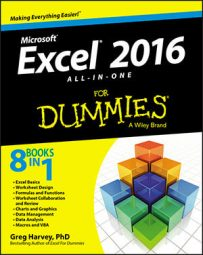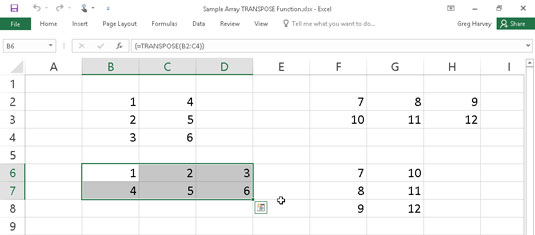##### Excel 2016 All-in-One For DummiesThe Excel 2016 reference functions on the Lookup & Reference command button's drop-down list on the Formulas tab of the Ribbon are designed to deal specifically with different aspects of cell references in the worksheet. This group of functions includes:

• ADDRESS to return a cell reference as a text entry in a cell of the worksheet

• AREAS to return the number of areas in a list of values (areas are defined as a range of contiguous cells or a single cell in the cell reference)

• COLUMN to return the number representing the column position of a cell reference

• COLUMNS to return the number of columns in a reference

• FORMULATEXT to return the formula referenced as a text string

• GETPIVOTDATA to return data stored in an Excel pivot table

• HYPERLINK to create a link that opens another document stored on your computer, network, or the Internet (you can also do this with the Insert→Hyperlink command)

• INDIRECT to return a cell reference specified by a text string and bring the contents in the cell to which it refers to that cell

• LOOKUP to return a value from an array

• OFFSET to return a reference to a cell range that's specified by the number of rows and columns from a cell or a cell range

• ROW to return the row number of a cell reference

• ROWS to return the number of rows in a cell range or array

• RTD to return real-time data from a server running a program that supports COM (Component Object Model) automation

• TRANSPOSE to return a vertical array as a horizontal array and vice versa

## Get the skinny on columns and rows

The COLUMNS and ROWS functions return the number of columns and rows in a particular cell range or array. For example, if you have a cell range in the spreadsheet named product_mix, you can find out how many columns it contains by entering this formula:

`=COLUMNS(product_mix)`

If you want to know how many rows this range uses, you then enter this formula:

`=ROWS(product_mix)`

You can use the COLUMNS and ROWS functions together to calculate the total number of cells in a particular range. For example, if you want to know the exact number of cells used in the product_mix cell range, you create the following simple multiplication formula by using the COLUMNS and ROWS functions:

`=COLUMNS(product_mix)*ROWS(product_mix)`

Don't confuse the COLUMNS (plural) function with the COLUMN (singular) function and the ROWS (plural) function with the ROW (singular) function. The COLUMN function returns the number of the column (as though Excel were using the R1C1 reference system) for the cell reference that you specify as its sole argument. Likewise, the ROW function returns the number of the row for the cell reference that you specify as its argument.

## Transposing cell ranges

The TRANSPOSE function enables you to change the orientation of a cell range (or an array). You can use this function to transpose a vertical cell range where the data runs down the rows of adjacent columns to one where the data runs across the columns of adjacent rows and vice versa. To successfully use the TRANSPOSE function, not only must you select a range that has an opposite number of columns and rows, but you must also enter it as an array formula.

For example, if you're using the TRANSPOSE function to transpose a 2 x 5 cell range (that is, a range that takes up two adjacent rows and five adjacent columns), you must select a blank 5 x 2 cell range (that is, a range that takes five adjacent rows and two adjacent columns) in the worksheet before you use the Insert Function button to insert the TRANSPOSE function in the first cell. Then, after selecting the 2 x 5 cell range that contains the data that you want to transpose in the Array text box of the Function Arguments dialog box, you need to press Ctrl+Shift+Enter to close this dialog box and enter the TRANSPOSE function into the entire selected cell range as an array formula (enclosed in curly braces).

Suppose that you want to transpose the data entered into the cell range A10:C11 (a 2 x 3 array) to the blank cell range E10:F12 (a 3 x 2 array) of the worksheet. When you press Ctrl+Shift+Enter to complete the array formula, after selecting the cell range A10:C11 as the array argument, Excel puts the following array formula in every cell of the range:

`{=TRANSPOSE(A10:C11)}`

The following figure illustrates the use of the TRANSPOSE function. The cell range B2:C4 contains a 3 x 2 array. To convert this 3 x 2 array in the cell range B2:C4 to a 2 x 3 array in the range B6:D7, follow these steps:

1. Select the blank cell range B6:D7 in the worksheet.

2. Click the Lookup & Reference command button on the Ribbon's Formulas tab and then choose the TRANSPOSE option from the button's drop-down menu.

Excel inserts =TRANSPOSE() on the Formula bar and opens the Function Arguments dialog box where the Array argument text box is selected.

3. Drag through the cell range B2:C4 in the worksheet so that the Array argument text box contains B2:C4 and the formula on the Formula bar now reads =TRANSPOSE(B2:C4).

4. Press Ctrl+Shift+Enter to close the Insert Arguments dialog box (don't click OK) and to insert the TRANSPOSE array formula into the cell range B6:D7 as shown here.Using the TRANSPOSE function to change the orientation of a simple array.

Clicking the OK button in the Function Arguments dialog box inserts the TRANSPOSE function into the active cell of the current cell selection. Doing this returns the #VALUE! error value to the cell. You must remember to press Ctrl+Shift+Enter to both close the dialog box and put the formula into the entire cell range.

If all you want to do is transpose row and column headings or a simple table of data, you don't have to go through the rigmarole of creating an array formula using the TRANSPOSE function. Simply copy the range of cells to be transposed with the Copy command button on the Home tab of the Ribbon. Position the cell cursor in the first empty cell where the transposed range is to be pasted before you click the Transpose option on the Paste command button's drop-down menu.